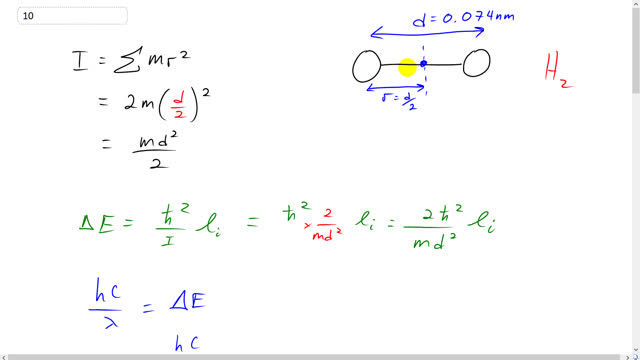## You are here

Question:

The equilibrium separation of H atoms in the $\textrm{H}_2$ molecule is 0.074 nm (Fig. 29–8). Calculate the energies and wavelengths of photons for the rotational transitions

1. l = 1 to l = 0,
2. l = 2 to l = 1, and
3. l = 3 to l = 2.Figure 29-8 Potential-energy diagram for the $H_2$ molecule; $r$ is the separation of the two H atoms. The binding energy (the energy difference between PE = 0 and the lowest energy state near the bottom of the well) is 4.5 eV, and $r_0 = 0.074 \textrm{ nm}$.

Giancoli, Douglas C., Physics: Principles with Applications, 7th Ed., ©2014. Reprinted by permission of Pearson Education Inc., New York.
The question will be visible after logging in, as required by Pearson Education Inc.

1. $\Delta E = 1.5 \times 10^{-2} \textrm{ eV, } \lambda = 82 \; \mu \textrm{m}$
2. $\Delta E = 3.0 \times 10^{-2} \textrm{ eV, } \lambda = 41 \; \mu \textrm{m}$
3. $\Delta E = 4.5 \times 10^{-2} \textrm{ eV, } \lambda = 27 \; \mu \textrm{m}$